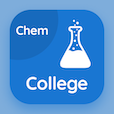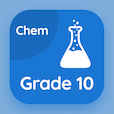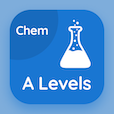Online College Courses

College Chemistry MCQs

College Chemistry MCQ - Topics

Practice Electron Radius and Energy Derivation Multiple Choice Questions (MCQ Quiz), Electron Radius and Energy Derivation quiz answers PDF to study college chemistry course for college chemistry online classes. Atomic Structure Multiple Choice Questions and Answers (MCQs), Electron Radius and Energy Derivation quiz questions for online colleges for science. Electron Radius and Energy Derivation Book PDF: energy of revolving electron, hydrogen spectrum, rutherford model of atom, electron distribution test prep for online college for teaching degree.

"The second orbit of hydrogen is far from the first orbit at about" MCQ PDF: electron radius and energy derivation App APK with two times, six times, four times, and nine times choices for online colleges for science. Learn electron radius and energy derivation quiz questions for merit scholarship test and certificate programs for accredited online colleges.

## MCQ on Electron Radius and Energy Derivation Quiz

MCQ: The second orbit of hydrogen is far from the first orbit at about

two times
six times
four times
nine times

MCQ: The square number of orbits is directly proportional to

shape of hydrogen
color of hydrogen
size of hydrogen

MCQ: The value of electron permittivity in Coulomb square per Joule per meter is

6*10^-12
9.34*10^-12
8.84*10^-12
5*10^-12

MCQ: The radius of hydrogen first orbit is

0.529 angstrom
2.11 angstrom
13.22 angstrom
4.75 angstrom

MCQ: Proton number is represented by

A
G
H
Z

### More Quizzes on College Chemistry Book